• Call Now

1800-102-2727•

# Lens formula, derivation, sign conventions, practice problems, FAQs

Lens is mainly of two types: convex lens and concave lens. The rays falling on convex lenses converge, so it is also called converging lens and the rays falling on concave lenses diverge, so it is called diverging lens. The image’s nature and location by these lenses depends upon the position of the object and the focal length of the lenses. The image may be real or imaginary for different positions of the object. The position of the image can be calculated by knowing the lens formula. Let's see what the lens formula is !

Table of content

• What is lens formula?
• Derivation of lens formula
• Sign conventions
• Practice problems
• FAQs

## What is lens formula?

Lens formula is the relation between the position of object, position of image and focal length of lens taking the optical center of lens as origin.

Mathematically it is given as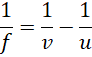Where,
u = Distance of the object from origin

v = Distance of the image from origin

f = Focal length of the lens

## Derivation of lens formula

Consider a thin convex lens of focal length F. An object of height h is placed at some point beyond 2F. Let the distance between the optical center and the object be u. The image is formed between F and 2F at a distance v from the optical center. The height of the image formed is h’.In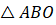and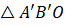,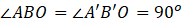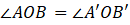(Vertically opposite angle)

Since all angles are the same , triangles are similar.

Hence,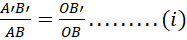Also in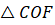and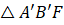,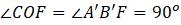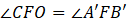(Vertically opposite angles)

Since all angles are the same, triangles are similar.

Hence,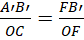Since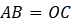, we get,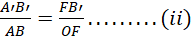From the equation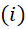and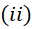, we get,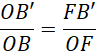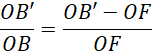By substituting the value with sign convention, we gets,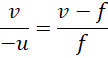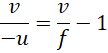This is known as lens formula. This is valid for both convex and concave lenses.

## Sign conventions

1. The optical center of the lens should be taken as the origin.
2. The principal axis should be the x-axis.
3. All the distances measured along the principal axis in the direction of the incident ray are taken as positive, while the distances measured against the direction of the incident ray are taken as negative.
4. Distances above the principal axis are taken as positive and below the principal axis are taken as negative values.

## Practice problems

Q. An object is placed perpendicular to the principal axis of a convex lens at a distance of 15 cm. If the focal length of a convex lens is 10 cm, find the nature and position of the image.

A. Given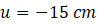and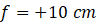Using lens formula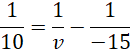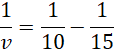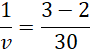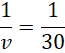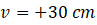Hence the image is at 30 cm from the lens and positive sign indicate, image is behind the lens and real.

Q.Where should the object be placed so that a real image behind the lens at a distance 30 cm can be formed by a converging lens of 15 cm focal length?

A. Given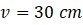and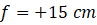Using lens formula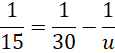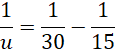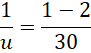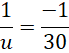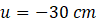Object should be placed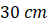in front of the lens.

Q. Keerti stands in front of the lens at a distance of 17 cm. If her image is formed in front of the lens at 10 cm, find the focal length and type of the lens.

A.Given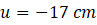and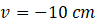Using lens formula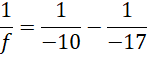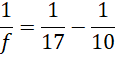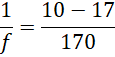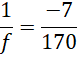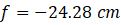(-ve) sign shows lens is diverging (concave lens) type.

Q. Where will the image be produced if an object is placed at a distance of 6 cm in front of a concave lens of focal length equal to 3 cm?

A. Given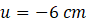and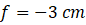By Applying lens formula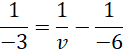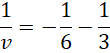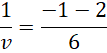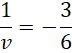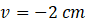Image will form at 2 cm in front of the lens.

## FAQs

Q. What are the assumptions for lens formula?

A.
1. The thickness of lenses should be less.
2. Lens has a small aperture.

Q. How does the lens formula differ from mirror formula?
A. Mirror formula and lens formula is given as

Q. Which principal focus is used in the lens formula for calculation?
A. Second principal focus is used for calculation.

Q. What are the applications of lens formula?
A. Len formula is used in locating the position and nature of the image, finding the focal length of unknown lenses etc.Talk to our expert
Resend OTP Timer =
By submitting up, I agree to receive all the Whatsapp communication on my registered number and Aakash terms and conditions and privacy policy